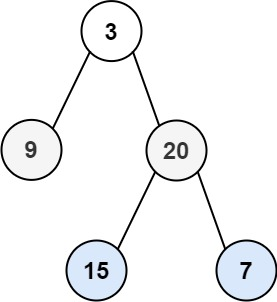## C# || How To Traverse Binary Tree Zigzag Level Order Using C#The following is a module with functions which demonstrates how to traverse binary tree zigzag level order using C#.

1. Zigzag Level Order – Problem Statement

Given the root of a binary tree, return the zigzag level order traversal of its nodes’ values. (i.e., from left to right, then right to left for the next level and alternate between).

Example 1:``` Input: root = [3,9,20,null,null,15,7] Output: [,[20,9],[15,7]] ```

Example 2:

``` Input: root =  Output: [] ```

Example 3:

``` Input: root = [] Output: [] ```

2. Zigzag Level Order – Solution

The following is a solution which demonstrates how to traverse binary tree zigzag level order.

This solution uses Breadth First Search to explore items at each level.

``` 2. Zigzag Level Order - Solution C# // ============================================================================ // Author: Kenneth Perkins // Date: Oct 29, 2021 // Taken From: http://programmingnotes.org/ // File: Solution.cs // Description: Demonstrates how to traverse a tree zigzag level order // ============================================================================ /** * Definition for a binary tree node. * public class TreeNode { * public int val; * public TreeNode left; * public TreeNode right; * public TreeNode(int val=0, TreeNode left=null, TreeNode right=null) { * this.val = val; * this.left = left; * this.right = right; * } * } */ public class Solution { public IList<IList<int>> ZigzagLevelOrder(TreeNode root) { var result = new List<IList<int>>(); var stack = new Stack<TreeNode>(); // Add root to stack if (root != null) { stack.Push(root); } var leftToRight = true; while (stack.Count > 0) { var queue = new Queue<TreeNode>(); var level = new List<int>(); // Add items at the top of the stack to the current level for (var itemCount = stack.Count; itemCount > 0; --itemCount) { var current = stack.Peek(); stack.Pop(); level.Add(current.val); queue.Enqueue(current); } // Add level to result result.Add(level); // Go through items in the queue and add them to the stack in the proper order for (var itemCount = queue.Count; itemCount > 0; --itemCount) { var current = queue.Peek(); queue.Dequeue(); // Add children to the stack // Left to right or Right to left order if (leftToRight) { if (current.left != null) { stack.Push(current.left); } if (current.right != null) { stack.Push(current.right); } } else { if (current.right != null) { stack.Push(current.right); } if (current.left != null) { stack.Push(current.left); } } } // Alternate order for the next level leftToRight = !leftToRight; } return result; } }// http://programmingnotes.org/ 12345678910111213141516171819202122232425262728293031323334353637383940414243444546474849505152535455565758596061626364656667686970717273747576 // ============================================================================//    Author: Kenneth Perkins//    Date:   Oct 29, 2021//    Taken From: http://programmingnotes.org///    File:  Solution.cs//    Description: Demonstrates how to traverse a tree zigzag level order// ============================================================================/** * Definition for a binary tree node. * public class TreeNode { *     public int val; *     public TreeNode left; *     public TreeNode right; *     public TreeNode(int val=0, TreeNode left=null, TreeNode right=null) { *         this.val = val; *         this.left = left; *         this.right = right; *     } * } */public class Solution {    public IList<IList<int>> ZigzagLevelOrder(TreeNode root) {        var result = new List<IList<int>>();        var stack = new Stack<TreeNode>();         // Add root to stack        if (root != null) {            stack.Push(root);        }         var leftToRight = true;        while (stack.Count > 0) {            var queue = new Queue<TreeNode>();            var level = new List<int>();             // Add items at the top of the stack to the current level            for (var itemCount = stack.Count; itemCount > 0; --itemCount) {                var current = stack.Peek();                stack.Pop();                 level.Add(current.val);                queue.Enqueue(current);            }             // Add level to result            result.Add(level);             // Go through items in the queue and add them to the stack in the proper order            for (var itemCount = queue.Count; itemCount > 0; --itemCount) {                var current = queue.Peek();                queue.Dequeue();                 // Add children to the stack                // Left to right or Right to left order                if (leftToRight) {                    if (current.left != null) {                        stack.Push(current.left);                    }                    if (current.right != null) {                        stack.Push(current.right);                    }                } else {                    if (current.right != null) {                        stack.Push(current.right);                    }                    if (current.left != null) {                        stack.Push(current.left);                    }                }            }            // Alternate order for the next level            leftToRight = !leftToRight;        }        return result;    }}// http://programmingnotes.org/ ```

QUICK NOTES:
The highlighted lines are sections of interest to look out for.

The code is heavily commented, so no further insight is necessary. If you have any questions, feel free to leave a comment below.

Once compiled, you should get this as your output for the example cases:

``` [,[20,9],[15,7]] [] [] ```